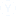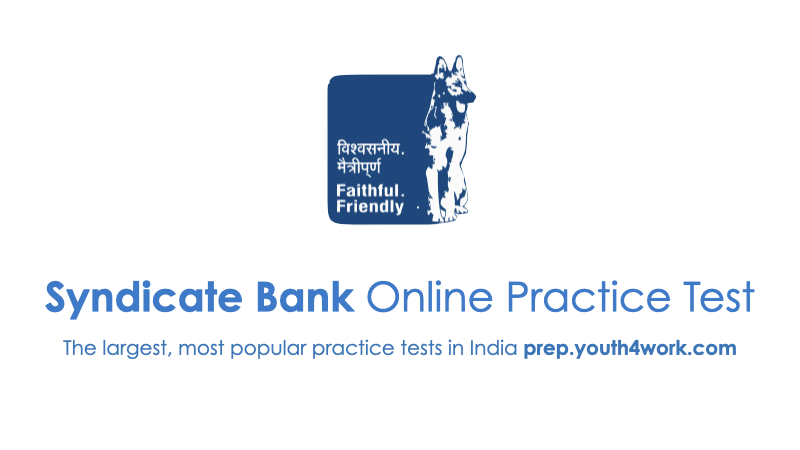# plz tell me what im doing to increase my quanti appti

solve my problem

Quantitaive Aptitude is divided into 5 parts:
1. Arithmetic (Includes Percentages, Simple-Compound Interest, Profit-loss discount, Ratios, proportions, mixtures allegations, time-speed-distance & time-work)
2. Algebra (Includes linear & quadratic equations, functions, logs, etc etc)
3. Geometry (Lines, angles, triangles, polygons, mensuration, 3-D geo etc etc)
4. Number System (includes classifications, surds, simplifications, remainders, cyclicity)
5. Modern Maths (Permutations, Combinations & Probability)

-1Other Related DiscussionsPractice Mock Test
syndicate bank